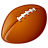# QlikView App Dev

Discussion Board for collaboration related to QlikView App Development.

Announcements
Action-Packed Learning Awaits! QlikWorld 2023. April 17 - 20 in Las Vegas: REGISTER NOW
cancel
Showing results for
Did you mean:Creator

## Expression Help -2

Hi All,

I'm working on an XIRR expression that I need some help. I created a variable for "Amount" where user can increase or decrease the value for particular date. I want to aggregate this variable amount to the XIRR calculation.  Please see attached Excel spreadsheet.

=XIRR(Amount,IRR_Date)

Many Thanks,

Frank

1 Solution

Accepted SolutionsLuminary Alumni

Hi,

Check this

=Num(XIRR(If(IRR_Date = Makedate(2015, 12, 31), Amount - Fabs(vIRR), Amount),IRR_Date), '#,##0.00%')

Regards,

Jagan.

9 RepliesLuminary Alumni

Hi,

Try like this

=XIRR(If(IRR_Date = Makedate(2015, 12, 31), vIRR, Amount),IRR_Date)

Regards,

Jagan.Creator
Author

Thanks ! Jagan,

Not close to the expected IRR % if 12/31/2015 decreased by 30,000,000 to 270,000,000. Did you check out my Excel spreadsheet manual calculation? For IRR calculation, I use this function XIRR(Amount, IRR_Date) both of the Amount and IRR_Date are in series of value and series of dates.Luminary Alumni

Hi,

Check this

=Num(XIRR(If(IRR_Date = Makedate(2015, 12, 31), Amount - Fabs(vIRR), Amount),IRR_Date), '#,##0.00%')

Regards,

Jagan.Creator
Author

Thank You Jagan!!! It works!!

How can I make it more dynamic if I have 2 different dates and 2 different variables?Luminary Alumni

Hi,

Get dates from Input box and replace this in the expression like below

=Num(XIRR(If(IRR_Date = vDateVariable1, Amount - Fabs(vIRR1),

(If(IRR_Date = vDateVariable2, Amount - Fabs(vIRR2),

Amount)),IRR_Date), '#,##0.00%')

Hope this helps you.

Jagan.Creator
Author

Thanks again. Will check it out.Creator
Author

Jagan,

Let me know if you can help.

I have excluded fields in my xirr calc.  I want to incorporate your expression with below. Many Thanks!

XIRR({<Company-={'*XYZ.*'},[Type]-={'Income:,'Expense:'}>}[Amount],[IRR_Date])

=Num(XIRR(If(IRR_Date = Makedate(2015, 12, 31), Amount - Fabs(vIRR), Amount),IRR_Date), '#,##0.00%')Luminary Alumni

Hi,

Try like this

=Num(XIRR(If(IRR_Date = Makedate(2015, 12, 31) AND Company NOT LIKE  '*XYZ*' AND [Type] <> 'Income:' AND Type <> 'Expense:', Amount - Fabs(vIRR), Amount),IRR_Date), '#,##0.00%')

Hope this helps you.

Regards,

Jagan.Creator
Author

Thanks Again!Community Browser